# 12.1AdjacencyMatrix: Representing a Graph by a Matrix

An adjacency matrix is a way of representing an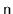vertex graph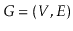by an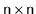matrix,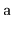, whose entries are boolean values.

    int n;
boolean[][] a;
n = n0;
a = new boolean[n][n];
}


The matrix entry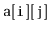is defined asThe adjacency matrix for the graph in Figure 12.1 is shown in Figure 12.2.

In this representation, the operations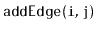,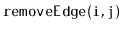, and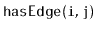just involve setting or reading the matrix entry:

    void addEdge(int i, int j) {
a[i][j] = true;
}
void removeEdge(int i, int j) {
a[i][j] = false;
}
boolean hasEdge(int i, int j) {
return a[i][j];
}

These operations clearly take constant time per operation.

Figure 12.2: A graph and its adjacency matrix.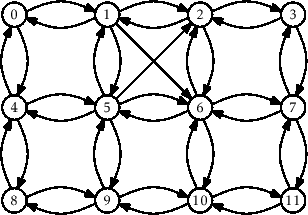0 1 2 3 4 5 6 7 8 9 10 11 0 0 1 0 0 1 0 0 0 0 0 0 0 1 1 0 1 0 0 1 1 0 0 0 0 0 2 1 0 0 1 0 0 1 0 0 0 0 0 3 0 0 1 0 0 0 0 1 0 0 0 0 4 1 0 0 0 0 1 0 0 1 0 0 0 5 0 1 1 0 1 0 1 0 0 1 0 0 6 0 0 1 0 0 1 0 1 0 0 1 0 7 0 0 0 1 0 0 1 0 0 0 0 1 8 0 0 0 0 1 0 0 0 0 1 0 0 9 0 0 0 0 0 1 0 0 1 0 1 0 10 0 0 0 0 0 0 1 0 0 1 0 1 11 0 0 0 0 0 0 0 1 0 0 1 0

Where the adjacency matrix performs poorly is with the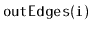andoperations. To implement these, we must scan allentries in the corresponding row or column ofand gather up all the indices,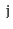, where, respectively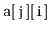, is true.

    List<Integer> outEdges(int i) {
List<Integer> edges = new ArrayList<Integer>();
for (int j = 0; j < n; j++)
return edges;
}
List<Integer> inEdges(int i) {
List<Integer> edges = new ArrayList<Integer>();
for (int j = 0; j < n; j++)
return edges;
}

These operations clearly take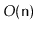time per operation.

Another drawback of the adjacency matrix representation is that it is large. It stores anboolean matrix, so it requires at least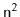bits of memory. The implementation here uses a matrix of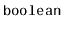values so it actually uses on the order ofbytes of memory. A more careful implementation, which packsboolean values into each word of memory, could reduce this space usage to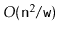words of memory.

Theorem 12..1   The AdjacencyMatrix data structure implements the Graph interface. An AdjacencyMatrix supports the operations
•,, andin constant time per operation; and
•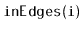, andintime per operation.
The space used by an AdjacencyMatrix is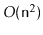.

Despite its high memory requirements and poor performance of theandoperations, an AdjacencyMatrix can still be useful for some applications. In particular, when the graph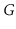is dense, i.e., it has close toedges, then a memory usage ofmay be acceptable.

The AdjacencyMatrix data structure is also commonly used because algebraic operations on the matrixcan be used to efficiently compute properties of the graph. This is a topic for a course on algorithms, but we point out one such property here: If we treat the entries ofas integers (1 for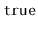and 0 for) and multiplyby itself using matrix multiplication then we get the matrix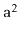. Recall, from the definition of matrix multiplication, that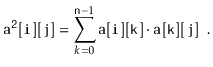Interpreting this sum in terms of the graph, this formula counts the number of vertices,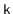, such thatcontains both edges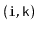and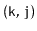. That is, it counts the number of paths from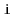to(through intermediate vertices,) whose length is exactly two. This observation is the foundation of an algorithm that computes the shortest paths between all pairs of vertices inusing only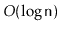matrix multiplications.

opendatastructures.org